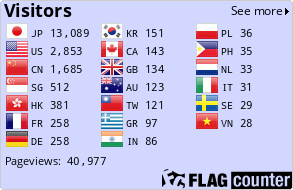Yu YANG@RIMS, Kyoto University

# Homepage of Yu YANG

Project Assistant Professor
Research Institute for Mathematical Sciences, Kyoto University
Kyoto 606-8502, Japan
e-mail: yuyang(at)kurims.kyoto-u.ac.jp

- Publications/Preprints
- Talks
- Conferences
- Žö‹Ć

## Publications

• On the existence of specialization isomorphisms of admissible fundamental groups in positive characteristic, [pdf].
To appear in Math. Res. Lett.

• On the averages of generalized Hasse-Witt invariants of pointed stable curves in positive characteristic, [pdf].
Math. Z. 295 (2020), 1-45. Online version

• The anabelian geometry of curves over algebraically closed fields of postive characteristic, [pdf].
To appear in RIMS Kokyuroku Bessatsu.

• Group-theoretic characterizations of almost open immersions of curves, [pdf].
J. Algebra 530 (2019), 290-325. Online version

• On the admissible fundamental groups of curves over algebraically closed fields of characteristic p>0, [pdf].
Publ. Res. Inst. Math. Sci. 54 (2018), 649-678.

• On the existence, geometry and p-ranks of vertical fibers of coverings of curves, [pdf].
RIMS Kokyuroku Bessatsu B72 (2018), 187-193.

• On the ordinariness of coverings of stable curves, [pdf].
C. R. Math. Acad. Sci. Paris, Ser. I 356 (2018), 17-26. Online version

• On the existence of non-finite coverings of stable curves over complete discrete valuation rings, [pdf].
Math. J. Okayama Univ. 61 (2019), 1-18.

• Degeneration of period matrices of stable curves, [pdf].
Kodai Math. J. 41 (2018), 125-153.

## Preprints

• Moduli spaces of fundamental groups of curves in positive characteristic I, [pdf].
• Raynaud-Tamagawa theta divisors and new-ordinariness of ramified coverings of curves, [pdf]. Submitted.
• Maximums of generalized Hasse-Witt invariants of pointed stable curves in positive characteristic, [pdf]. Submitted.
• Finite quotients of fundamental groups and moduli spaces of curves in positive characteristic, [pdf]. Submitted.
• The combinatorial mono-anabelian geometry of curves over algebraically closed fields of positive characteristic I: combinatorial Grothendieck conjecture, [pdf]. Submitted.
• Tame anabelian geometry and moduli spaces of curves over algebraically closed fields of characteristic p>0, [pdf]. Submitted.
• p-rank and semi-stable reduction of coverings of curves, [pdf]. Submitted.

• Moduli spaces of fundamental groups of curves in positive characteristic III, in preparation.
• Moduli spaces of fundamental groups of curves in positive characteristic II, in preparation.
• On the averages of p-rank of generic curves in positive characteristic, in preparation.
• The combinatorial mono-anabelian geometry of curves over algebraically closed fields of positive characteristic II: reconstructions of semi-graphs of anabelioids via open continuous homomorphisms, in preparation.visits to this page since August 12, 2017.